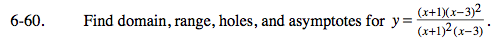### Home > CALC > Chapter 6 > Lesson 6.2.1 > Problem6-60

6-60.Horizontal asymptotes, if any, can be found by exploring limit of f(x) as x→∞ and limit of f(x) as x→−∞.
If either, or both, are constants, then the constant(s) is/are the horizontal asymptote(s).

Vertical asymptotes and holes occur where the denominator equals 0. If the zero on the denominator 'cancels out', then that is the x-value of the hole. Find the y-value too. If the zero in the denominator remains, then that is the vertical asymptote.

Now that you have found all holes and asymptotes... Use the x-values of all holes and vertical asymptotes to restrict the domain. Also consider endpoint(s). Use the y-values of all holes and horizontal asymptotes to restrict the range. Also, consider endpoint(s).